# The Most Important Irrational Constants Joined Together

Believe it or not, but it took me 4 years to discover that pi2 ≈ phi2 + e2. In this article I'm going to explain what this connection means.

Since the beginning of my adventure with Theory of Thought, I’ve always held that Pi, Phi, and e were fundamental concepts in my theory - particularly in a philosophical sense. But until I discovered this geometry I had had no idea how deeply interwoven these constants truly were.

There are few reasons you won't find any references to this geometry online, including:

1) most people haven’t tried the calculation,
2) the values in the equation require considerable truncation (considered erroneous by mathematicians)
3) its significance is too puzzling to be widely accepted

Since I already had a theory about these three irrational constants, it was striking to discover that this theory also had a geometrical perspective that might explain the hidden framework holding a mind together.

Thought Theory explains that mindspace is geometrical, because it's a mathematical architecture that maintains patterns.

Pi, Phi, and e are found in a majority of equations used in classical and quantum mechanics; and through their relations, they are also vital in calculating the structure of the hyper-dimensional spaces within the mind.

They are the most important numbers in the universe, and Thought Theory merges these numbers, their meanings, and their mathematical concepts into a single model describing the basic process of mindspace.

And it reveals something extra-ordinary.

Consider that the equation binding the three constants is none other than the Pythagorean theorem, the most respected equation in nature.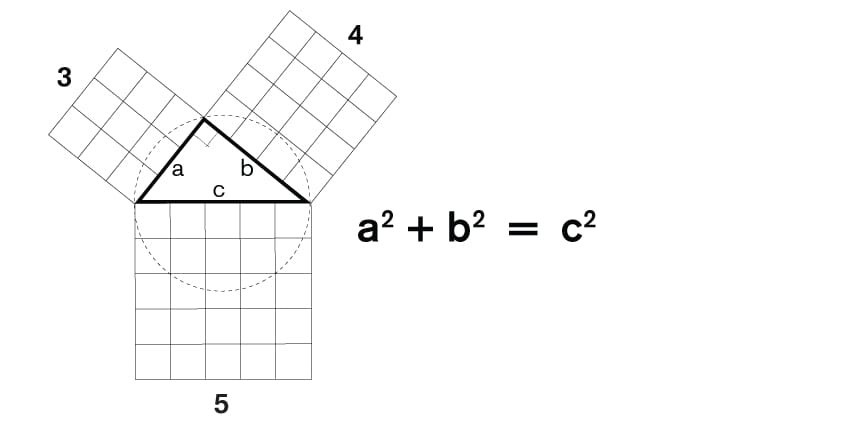This equation is unique because it's used to calculate distances between any two points on a spatial plane.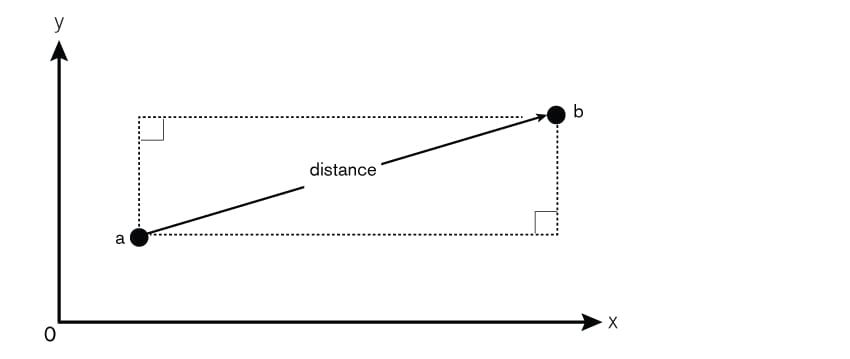And within our brains and our minds, our thoughts must also be organized across distances and spaces.

But in contrast to most linear measurements, the distances within a mind are calculated in rotations or cycles.

But why would the connections between pi, phi, and e be significant?

Because conceptually:

PI = Circular Perimeter
PHI = Expansion
e = Growth

Combined together, these three constants open up a region within the abstract space. Their function is to architect a hyper-dimensional area that can be populated with relationship patterns (hierarchical, union, network, system, and gear). The patterns are assembled into 'spaces' and the interaction between these spaces leads to intelligent thought and symbolism.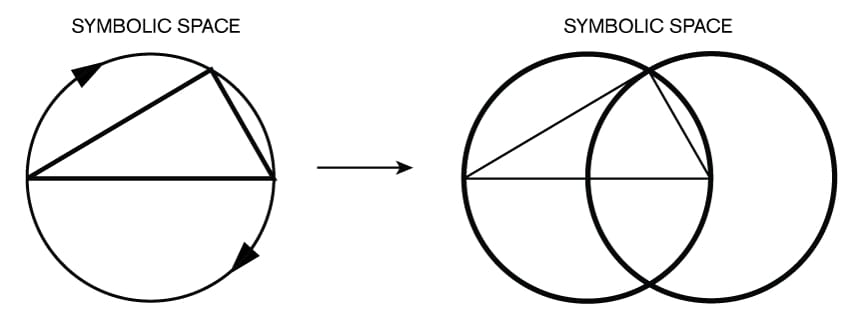Mindspace is based on the Pythagorean Dyad: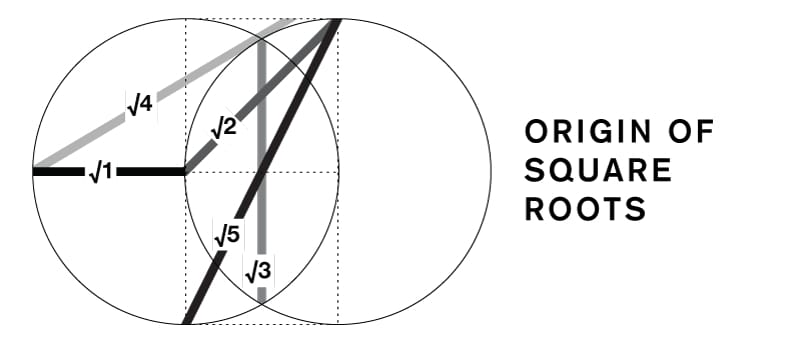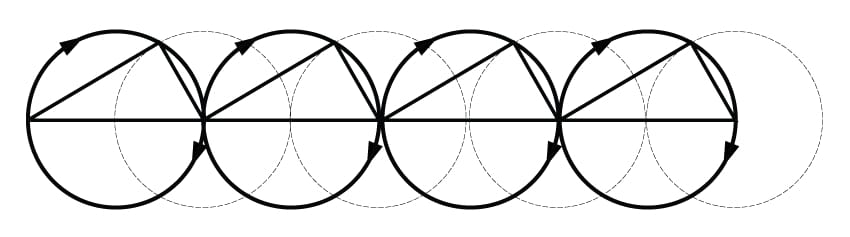'In quantum field theory, the fabric of space is visualized as consisting of fields, with the field at every point in space and time being a quantum harmonic oscillator, with neighboring oscillators interacting.' - Wikipedia

... so each 'field' in mindspace is an oscillator governed by a Tetractys inside its Vesica Piscis: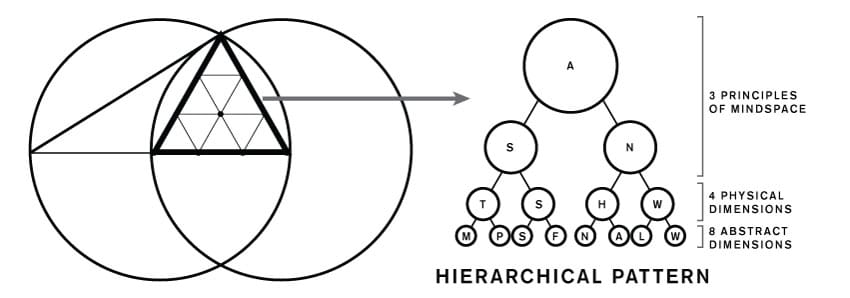The Tetractys represents the root of a hierarchical pattern within mindspace.

Each symbol is under the control of the fundamental triangle, which can be divided into two sections: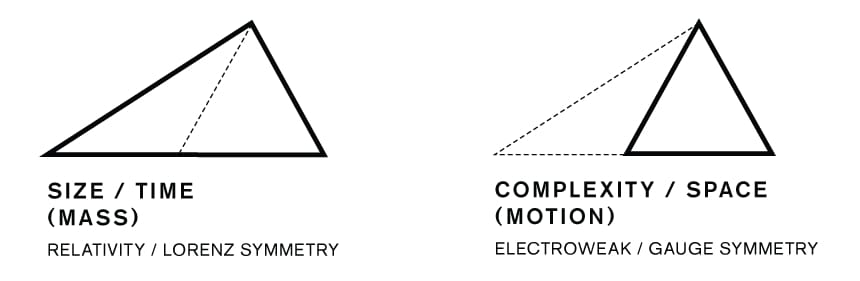And each triangle can be visualized as a bridge connecting the concepts of Time and Space: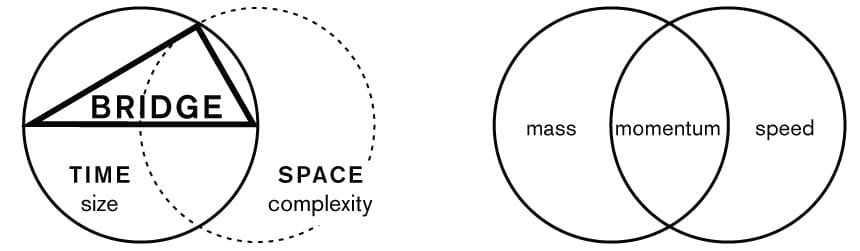PI, PHI, and e are root concepts that manage the exchange of information (ie. patterns) between pockets of time in a mindspace.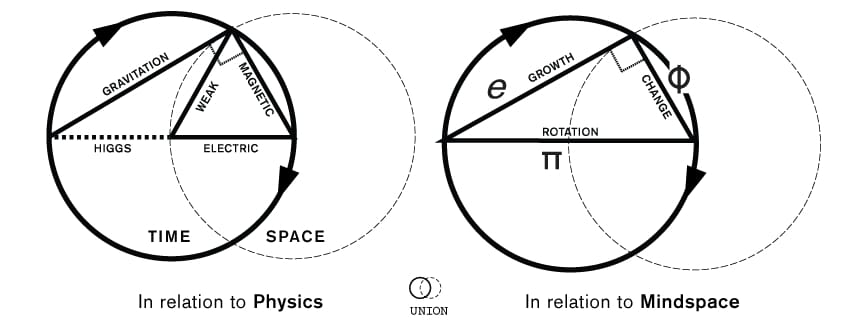And should be thought of as an ideal mechanism that generates natural forces which affect both symbols in a mindspace and arrangements of matter in a physical space, simultaneously.